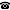# 2014 seminar talk: On von Neumann's Theorem and Borel complexity of unitary equivalence modulo compact perturbations of self-adjoint operators

Talk held by Hiroshi Ando (Erwin Schrödinger Institute, Vienna, Austria) at the KGRC seminar on 2014-01-30.

### Abstract

The celebrated Weyl-von Neumann perturbation theorem asserts that every self-adjoint operator can be turned into a diagonal operator by an arbitrarily small compact (in fact Hilbert-Schmidt) perturbations. As a corollary, von Neumann obtained the following result: for bounded self-adjoint operator $A$,$B$, the following conditions are equivalent:

(1) $uAu^*+K=B$, where $u$ is a unitary and $K$ is a compact opeartor.

(2) $A$ and $B$ have the same essential spectrum.

Here, the essential spectrum of $A$ is the set of all points in the spectrum of $A$ which is either an eigenvalue of infinite multiplicity or an accumulation point in the spectrum. Therefore, the essential spectrum is the complete invariant for the classification problem of bounded self-adjoint operators in the sense of (1).

Since Weyl-von Neumann theorem works for general unbounded self-adjoint operators, it is interesting to consider whether von Neumann's theorem still holds for unbounded operators. However, many examples indicates us that there is very little hope to find a complete and invariant $I(A)$ (assigned in a computable way) for each self-adjoint $A$ such that for $A,B$ $uAu^*+K=B$ holds for some $u$,$K$ iff $I(A)=I(B)$.

The purpose of our work is to justify this feeling by showing:

(a) It can be deduced from von Neumann's characteriztion that the classification of bounded self-adjoint opeartors up to unitary equivalence modulo compacts is smooth.

(b) The same type of equivalence relation for unbounded self-adjoint operatorss does not admit classification by countable structure, although the associated orbit equivalence relation is not turbulent.

This is a joint work with Yasumichi Matsuzawa (Shinshu University).

Kurt Gödel Research Center for Mathematical Logic. Währinger Straße 25, 1090 Wien, Austria.+43-1-4277-50501. Last updated: 2010-12-16, 04:37.